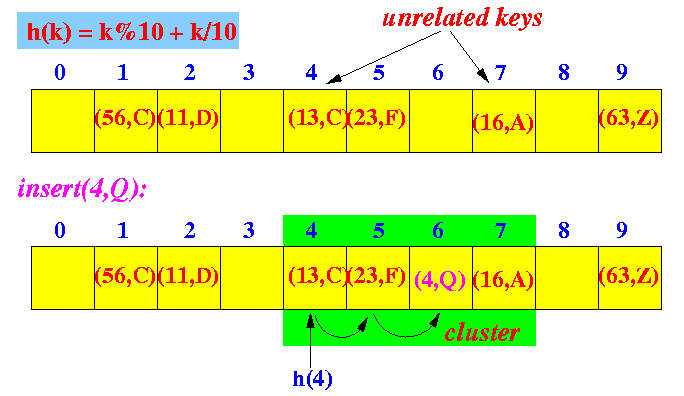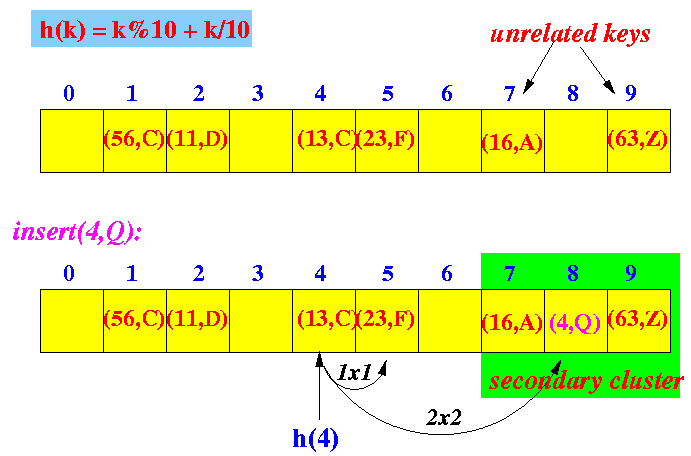### Open Addressing: Handling collision in hashing

 In Open address, each bucket stores (upto) one entry (i.e., one entry per hash location/address) When the hash location is occupied, a specific search (probe) procedure is invoked to locate the searched key or an empty slot

• Example probing scheme: Linear Probing (or Linear Addressing)

• Linear Probing:

 When a bucket i is used, the next bucket you will try is bucket i+1 The search can wrap around and continue from the start of the array.

• Input keys: (the values associated with the keys are omitted for brevity)

 18, 41, 22, 44, 59, 32, 31, 73 Hash array size: 13 Hash function: h(k) = k % 13

• Insertions:

• insert key 18: 18 % 13 = 5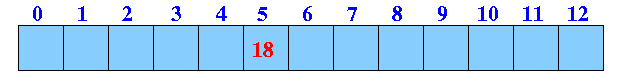• insert key 41: 41 % 13 = 2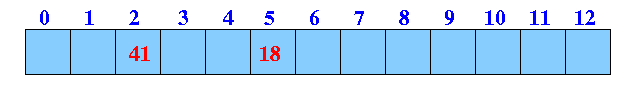• insert key 22: 22 % 13 = 9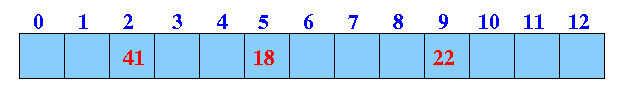• insert key 59: 59 % 13 = 7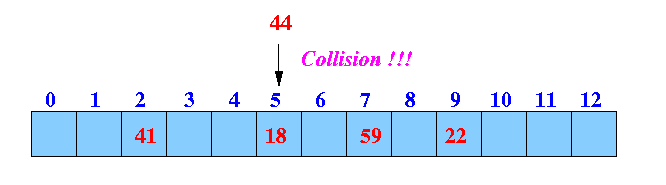• insert key 44: 44 % 13 = 5 ------ collision !!!Solution: search (= probe) for an empty slot in hash table: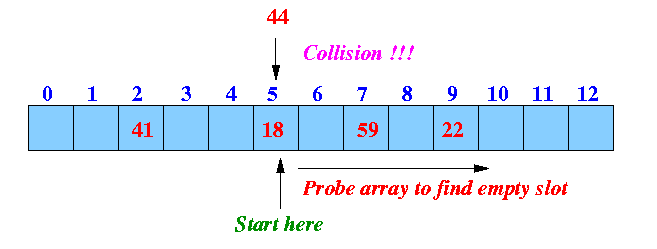Simplest probing procedure: linear probing --- look in next slow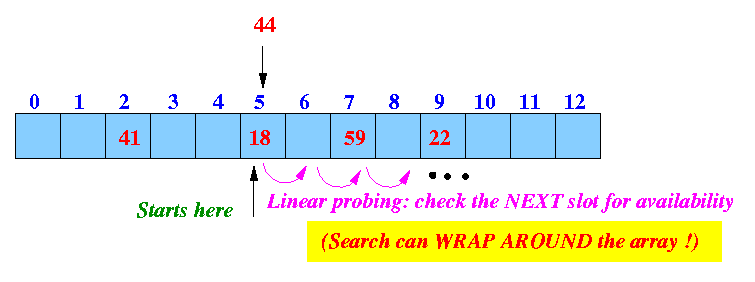Result: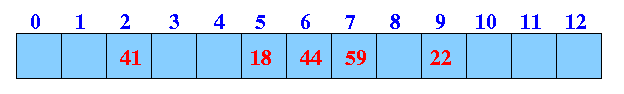Note:

• Keys that hash to the same hash bucket (= array element) will always be clustered together (= occupy consecutive array elements(

• But, unrelated keys can cluster !!!

Example:

 The unrelated key 59 and keys 18 and 44 has formed a cluster

• insert key 32: 32 % 13 = 6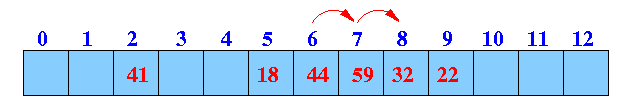Note:

• Bucket 6 is used

 We keep looking for an empty bucket to store the new key We found an (first) empty slot in bucket 8

• BTW, the cluster gets bigger (snow ball effect :-))

• insert key 31: 31 % 13 = 5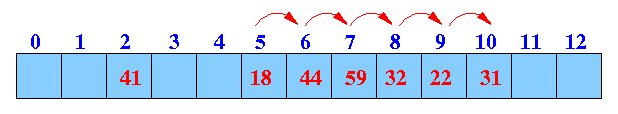Note:

• Bucket 5 is used

 We keep looking for an empty bucket to store the new key We found an (first) empty slot in bucket 10

• insert key 73: 73 % 13 = 8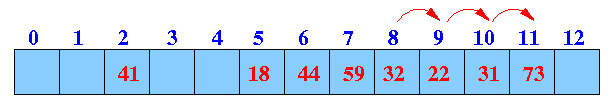Note:

• Bucket 8 is used

 We keep looking for an empty bucket to store the new key We found an (first) empty slot in bucket 11

• Pseudo code for linear probing:

 ``` start = HashValue( key ); // Start looking in the Hash location search_i = start; // Start here while ( bucket[sreach_i] != EMPTY /* not empty */ && "search did not wrap around" ) { search_i = (search_i + 1) % N; // Keep looking in the NEXT hash bucket } ```

• Notice:

 Increased occupancy level will increase the likelihood of collisions

• Lookup procedure in Open Addressing

• Lookup procedure for the key k

• i = hash the key k to find the bucket index b

• Starting at bucket b, look for the key k using the same probing procedure

• There will be 3 possible outcomes:

• You find the key k:

 In this case, you can perform the operation on the entry. E.g.: return the value, update the value of delete the entry

• You find an empty slot before you find the key k

 Then the key k is not stored in the hash table You return not found

• The search wrapped around (you end up where you started):

 Then the hash table is completely filled up and the key k is not stored in the hash table You return not found

• Example: get(31)

1. get(31): find the hash index (location)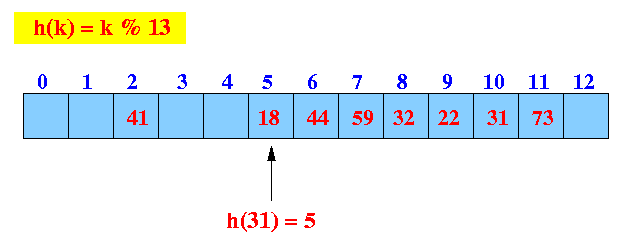Start looking at location 5

2. Linear probe procedure: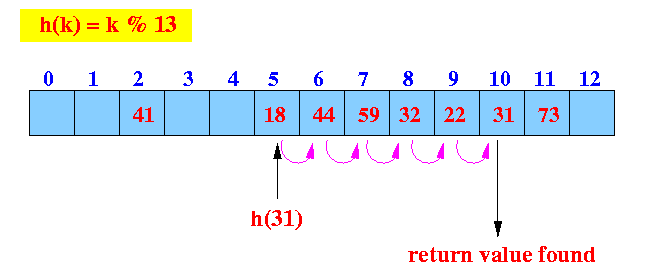We found the key (31) ==> return found.

• Example: get(19)

1. get(19): find the hash index (location)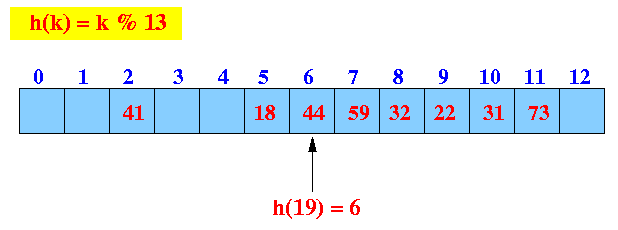Start looking at location 6

2. Linear probe procedure: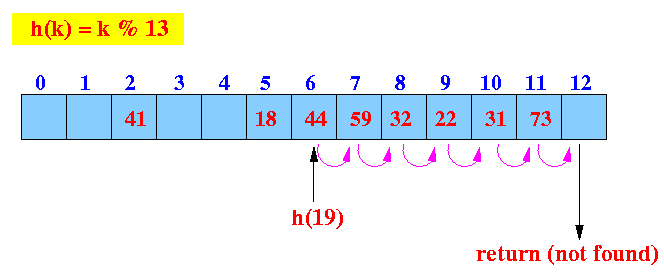We reach an empty slow before finding the key 19.

• Example: get(19) in a full hash table

1. get(19): find the hash index (location)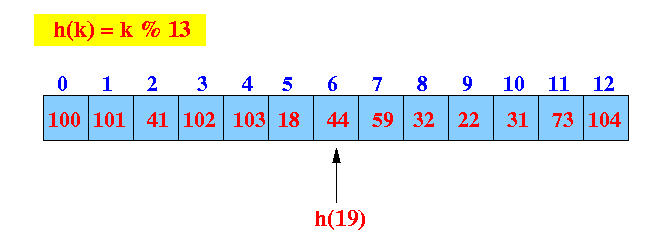Start looking at location 6

2. Linear probe procedure: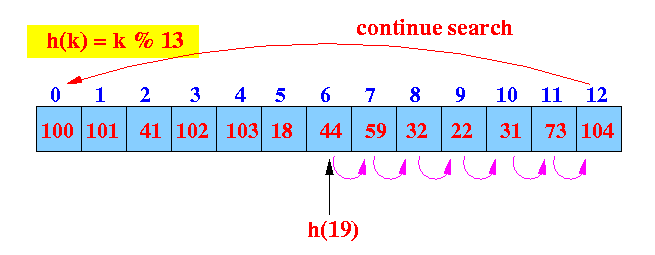We reach the end, the search continues from the start of the array: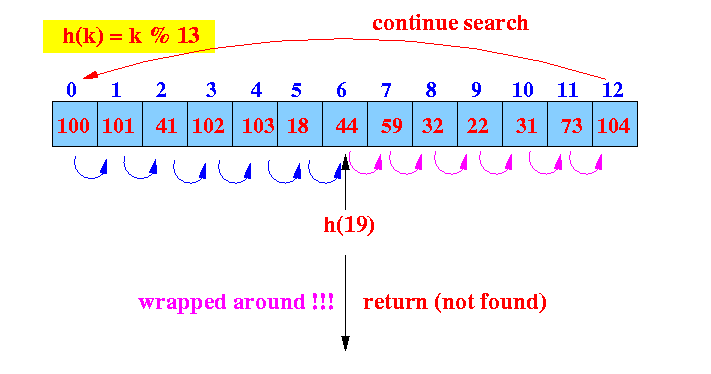The search has wrapped around complete ==> key is not in the hash table.

• Other kinds of probing procedures:

• Commonly used search procedures in Hashing:

• Linear probing:

• The location for the ith probe is hashIndex + i

Graphically: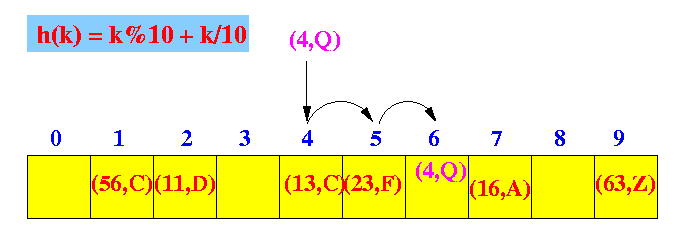• The location for the ith probe is hashIndex + i2

Graphically: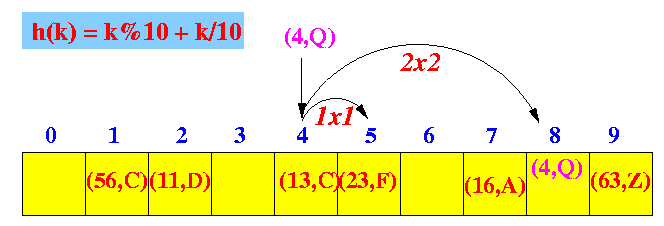• Double hashing:

• Uses 2 different hash functions: h(k) and h2(k):

 h(k) is used to find the hash location (hashIndex) h2(k) is used to probe

• The location for the ith probe is hashIndex + i × h2(k)

Graphically: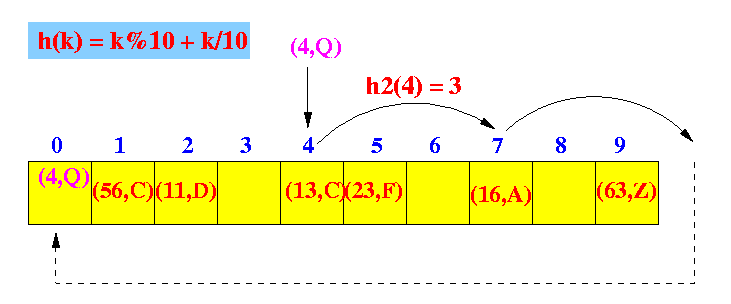• The secondary hash function:

• Cannot produce zero as hash result !

• Commonly used one:

 h2(k) = q − (k % q)         (q = a prime number < N)

• Pro's and con's:

• Linear probing:

• Simple to implement

• But can create clusters (series of occupied cells of unrelated keys)

Example: